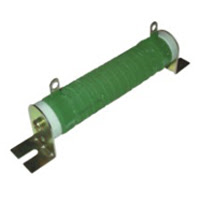## Thursday, August 27, 2009

### Resistors Part 1 - Use and Properties

Now that you have learned the basic concept of Ohm's Law, we can now proceed in discussing the use, properties, and construction of resistors. All you can learn it here in Electrical Engineering.

Before we continue our study of circuits, we need to know more about resistance and resistors though we have touched it a little on my previous post. But its just a review. Today, it would be a bit deeper.

We know that there is a certain amount of resistance in all electrical equipment which we use. Sometimes, this resistance is not enough to control the flow of current to the extent desired. If you did not get my point here, let's have a few example of this. I will going to give an example by illustration as what had shown below. The circuit shown below, a switch and a current limiting resistor are used to control the flow of current through the motor. When starting a motor, the switch is kept open and the resistance is thereby added into the circuit to control the flow of current. After the motor has started, the switch is then closed in order to bypass the current limiting resistor.There are wide variety of resistors, some of them have fixed values and some are variables.

What resistor is made up of?

Resistors are made up of special resistance wire, graphite (carbon) composition, or of metal film. Wire wound resistors are usually used to control large currents, while carbon resistors controls current which are relatively small.Vitreous enameled wire-would resistors are constructed by winding resistance wire on a porcelain base, attaching the wire ends to metal terminals, and coating the wire and base with powdered glass and bake enamel to protect the wire and conduct heat away from it.

Fixed wire wound resistors with a coating other than vitreous enamel are also used. The example below is the example of this one.Wire-wound resistors may have fixed taps, which can be also used to change the resistance value in steps, or sliders, which can be adjusted to change the resistance of any fraction of the total resistance. The picture below is the example of this one.Precision wound resistors of manganin wire (a special wire that does not change resistance very much with high temperature) are used where the resistance value must be very accurate, such as in test instruments. The picture below is the example of this one.Carbon resistors are used for low current applications. They are made from a rod of compensated graphite (carbon) that is mixed with clay and binders. By varying the amount of each component, it is possible to vary the resistance values obtained over a very wide range. Two lead wires are called pigtails are attached to the end of the resistance rod, and the rod is embedded in a ceramic or plastic covering, leaving the pigtails protruding from the ends. Take a look for a sample below.You will find other type of resistor called a deposited film resistor used for special applications. These resistors are made by depositing a thin film of resistance metal or carbon on a ceramic core and then coating the resistor with either a ceramic or enamel protective coating. In many cases you will find that these resistors have radial leads meaning the leads come off at right angles to the body of the resistor. In some cases the deposited film is laid down on the core as spiral, similar to winding a wire around the tube, in order to increase the length of the resistance element without making the resistor too long. The example of this one was shown below.

Resistor Tolerance and Values

Let's consider this topic before we go on the color code for resistors. You need to find out the something about resistor tolerances and something about the preferred values of resistance that you will find in the circuits. Special resistors may have tolerances of as little as 1% , 0.1% or even 0.01% but most resistors that you will see have much greater tolerances. Large wire-wound resistors usually have tolerances of 10% or 5%. Carbon resistors are available in 20%, 10% and 5% tolerances.

So what are those tolerance mentioned above means? Let's take an example...

If you had a 10 kilohm resistor with a tolerance of 20%, the actual value of the resistor could anywhere from 10 kilohm - 10 kilohm (0.20) = 8 kilohm to 10 kilohm + 10(0.20) = 12 kilohm. That is how you will going to use the tolerance given for a specific resistor.

You will wonder how many different resistance values you can get for a resistor. It depends on the tolerance. Considerations such as this have led to the establishment of a set of preferred values of resistance in each tolerance where the highest tolerance of one value is about equal to the lowest tolerance of the next highest value. The table of preferred resistance was shown below. Later, you will find that resistors are available in different power ratings as well.

The numbers on the chart above show only the first two digits. Thus, it means for example, 33 means that 3.3, 330, 3.3 kilohm, 330 kilohm and 3.3 megohm resistors are available.

On my next post I will show you how the resistor color code is being used for carbon resistors and how the resistance is being measured.

I will tee off shortly. Stay more here in Learn Electrical Engineering for Beginners.

Cheers!